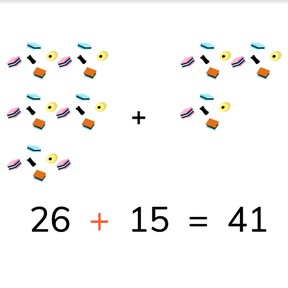No account needed.8,000 schools use Gynzy92,000 teachers use Gynzy1,600,000 students use Gynzy

## General

Students learn to add to 50 making tens with second addends that are greater than ten.

## Relevance

It is important to be able to add to 50, to be able to join larger amounts together.

## Introduction

Ask students to use the number line to calculate the total of the addition problem. You can ask them to count intermediate steps as well.

## Development

Discuss with students the importance of being able to add to 50. The lesson goal is then presented visually, in the abstract, and as story problems. Select the learning style that best suits your classroom by using the menu in the bottom right of the page. In the visual method, objects are first counted as separate groups, and then counted together. Show that with 19, you first add 10 more to reach 29, and then make tens with the remaining 8, to reach 30, and add the final 7 to reach the total of 37. Practice the next problem with the students. Check that students are able to solve using the visual method. With the abstract, different ways of calculation are explained. First demonstrate using the number line to add, showing how you first add the tens, and then the ones. Next using the number line, show how adding the tens, and the tens and the ones moves on the number line. Show two more ways in which you can calculate a total without the number line in the abstract. For story problems, the steps to solve a story problem are reviewed and then story problems are given to solve with the class.

Check that students understand addition to 50 making tens with second addends greater than 10 by asking the following questions:
- Why is it useful to add to 50?
- How do you calculate an addition problem to 50?

## Guided practice

Students first start with an addition problem with visual support. Then they are asked to solve an abstract, and finally they are given story problems to calculate.

## Closing

Check that students have understood the instruction by asking them how they calculate an addition problem to 50. Emphasize that every student will have a different favorite form of calculation and that one method is not superior to another. In the final exercise, students may select a ball. The color of the ball matches a number with which it is linked. Students may uncover a ball per turn. If a number is revealed, they must be added to the number on the left with the same color.

## Teaching tips

Students who have difficulty adding to 50 can first practice with problems to 30. Give them manipulatives like blocks, erasers, etc to help visually support the addition process. Practice recognizing which two addends make tens, like 7+3, 6+4, 8+2, etc. You can then expand to numbers that make tens and go on, like 9+2, 8+3, etc. Students can also be supported by using the number line.

## Instruction materials

Optional: Manipulatives and number line

### The online teaching platform for interactive whiteboards and displays in schools

• Save time building lessons

• Manage the classroom more efficiently

• Increase student engagement